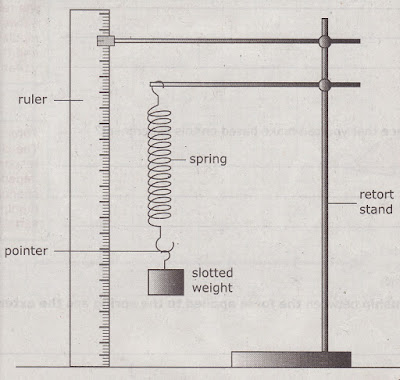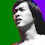## Wednesday, May 12, 2010

### [Science PMR] Exam Pointers I

A group of students carried out an experiment, using a spring and a set of slotted weights as shown in the diagram below, to investigate the relationship between the length of the spring and the weight.Number of slotted weights Extension of spring / cm 1 10 2 20 3 30 4 40 5 50
Table 1
1. State the variables that are involved in the experiment

• Manipulated variable => Number of slotted weights.
Common error: Students only write "weights". You must write out the answer in full.

• Responding variable => Extension of the spring.
Common error: Students only write "spring". You must write out the answer in full.

• Constant variable => The type of slotted weight/type of spring.
Common error: "Retort stand" is not acceptable. The answer should be the variable that when changed will affect the reading.

Tutor's comment: Look at the table 1, the first column is the manipulated variable and the second column is the responding variable.

2. What is the aim of this experiment?
Answer: To investigate the relationship between the number of slotted weights and the extension of the spring.

Tutor's comment: It is to investigate the relationship between the manipulated variable and the responding variable.

3. What is the inference that you can make based on this experiment?
Answer: The extension of the spring depends on / is determined by the number of slotted weights.

Tutor's comment: The changes in the responding variable depends on the changes in the manipulated variable.

4. What is the relationship between the force applied to the spring and the extension of the spring?
Answer: When the force applied to the spring increases, the extension of the spring increases.

5. Based on the data, draw a graph to show the relationship between the length of the spring and the number of slotted weights.Tutor's comment: Use a pencil when drawing the graph. If the question did not specify which type of graph to draw, draw a line graph.

6. Predict the length of the spring if 6 slotted weights are used.

Tutor's comment: Draw the corresponding lines on your graph and get the reading from the graph.

7. What conclusion can be drawn from the experiment?
Answer: When the number of slotted weights increases, the extension of the spring also increases.

Tutor's comment: The conclusion will be the same as your hypothesis, that is "When the manipulated variable increase/decreases, the responding variable will increase/decrease."

1.«°´¯*PoWKilLeR«°´¯*Haha*¯`°»PoWKilLeR«°´¯*Have a good day!*¯`°»
*Haha*¯`°»«°´¯*Haha*¯`°»PoWKilLeR«°´¯*Haha*¯`°»
PoWKilLeR«°´¯* good day!!*¯`°»
*Haha*¯`°»«°´¯*Have a good day!*¯`°»
«°´¯*PoWKilLeR«°´¯*Haha*¯`°»
*Haha*¯`°»

2.CAKGU MACAM MANA NAK Manipulated variable ,Responding variable,Constant variable

3.OHH thanks teacher :)

------------------
http://goalpooper.blogspot.com

## Nota Terkini

• - Sumber-sumber tenaga yang terdapat di dunia ini adalah boleh dikelaskan kepada dua kumpulan, iaitu: 1. Sumber tenaga yang boleh diperbaharui. 2. Sumb...
• - *Hukum-hukum Indeks – Hukum 1* *am x** an= am+n* dimana *a*, *m* dan *n* adalah nombor nyata. *Pembuktian Hukum 1* *Contoh 1* Cari nilai bagi yang ber...
• - Formula am sabun dapat diwakili dengan RCOONa untuk garam natrium atau RCOOK untuk garam kalium dengan R sebagai kumpulan alkil yang berantai panjang. Co...
• - *Kepupusan haiwan* (animal extinction) bermaksud *lenyapnya* atau *sudah tiada lagi* sesuatu haiwan itu di muka bumi ini. Kewujudan haiwan-haiwan tersebut...
• - Apabila suatu voltan, *V*, dikenakan merentasi katod dan anod suatu senapang elektron, tenaga elektrik yang dibekalkan kepada setiap elektron ialah *eV*, d...